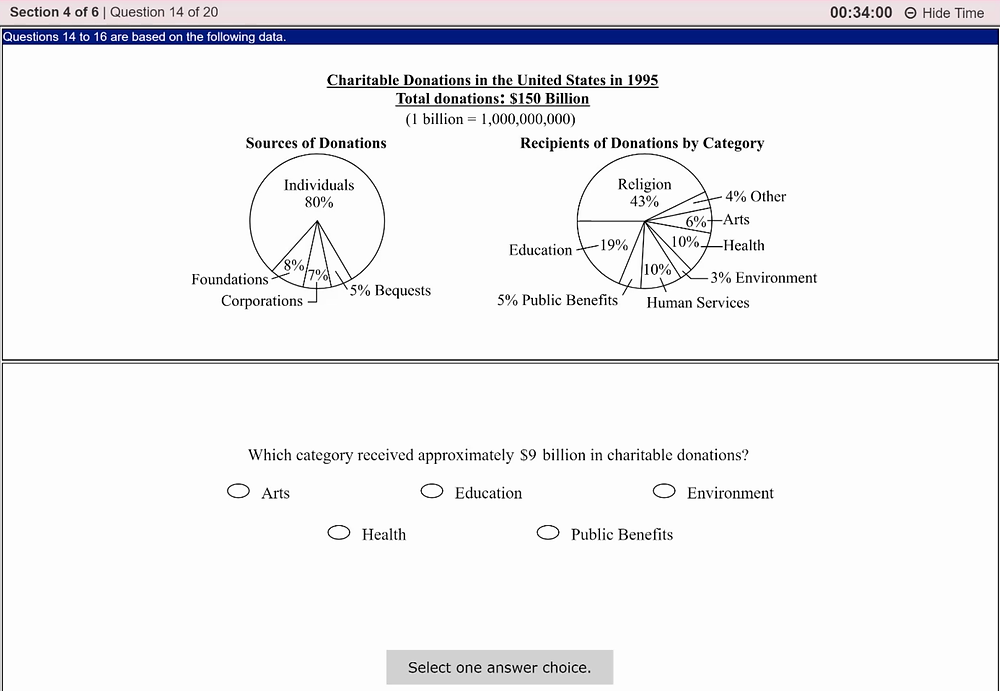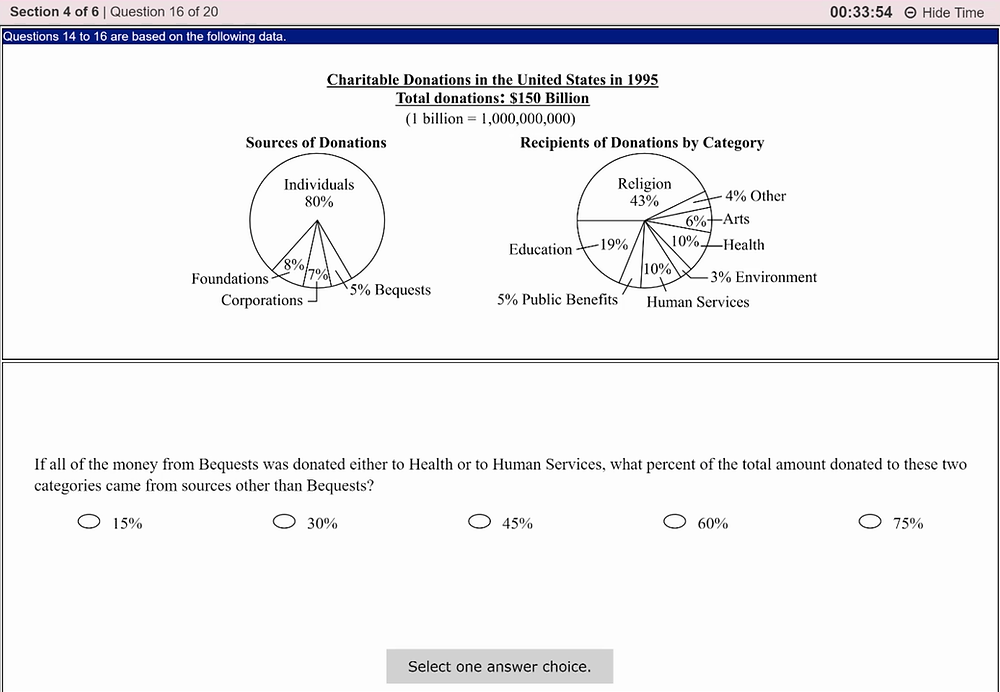# A Full Set of Hard Quant and Analysis

This post shares a full hard section of GRE Quant in a real at-home test, with keys and analysis. (sadly, questions 1 and 18 are missed...)

You can try these questions in no more than 35 minutes.Key: C

Analysis: You must know: (a-b)2=a2-2ab+b2, so quantity A=x2-1.4x+0.49, quantity B=0.49-1.4x+x2. QA-QB=0, so we choose C.Key: B

Analysis: For this question, we must know two laws of triangles. 1, the sum of the degree of three corners is 180. 2, in the graph, x+y=3x. So, we can have the following formula:

x+y+0.75y=180

x+y=3x

The solution is: x=40Key: B

Analysis: This is a rather simple algebra question. Quantity A=x2+2y2+3x+4y, Quantity B=y2+2x2+3y+4x. QA-QB=(y2-x2)+(y-x). Since x>y>0, (y2-x2)<0 and (y-x)<0. So, QA-QB<0, QB is greater.Key: A

Analysis: For this question, you really don't have to know which number is the median of the first 999 even or odd number. By asking the median of the first 999 even/odd integers, it is the same as asking which is the 1st even and odd integer. The 1st positive even integer is 2 and the 1st positive odd integer is 1. So, QA>QB.Key: D

Analysis: The easiest way to solve this question is by enumerating all three-digit integers beginning with 5 and ending with 8 and also multiple of 7. We have the following numbers: 518, 525, 532, 539, 546, 553, 560, 567, 574, 581, 598. Only 518 and 598 comply with the integer described in the question. Thus, the correct answer is D.Key: B

Analysis: Suppose the area of Hopetown is H and the area of the entire Lewis County is L. This question tells us that H=20%(L-H), and Quantity A=H/L. By simply solving the formula, we know that H/L=1/6, which is less than 18%, so the correct answer is B.Key: D

Analysis: This question is essentially a matter of positive and negative. If x is negative and y is positive, then xy must be less than zero, which is less than QB. But, if x and y are both negative, and we suppose x=-100, y=-100, then xy=+10000, which is greater than QB. So the correct answer is D.Key: E

Analysis: Radius=r, circumference=c, c=2πr, area=πr2, so the ratio of area of K and P is the square of the ratio of their circumference, which is 9:4.Key: E

Analysis:

0.6x-320>100

x>700Key: A

Analysis: There are totally 24 kinds of numbers formed in the way suggested in the question (4*3*2*1=24). If the number is to be greater than 3000, the thousands digit can only be 3 or 4. So, we have 2 possibilities in thousands digit and this leaves us 3 possibilities for hundreds and 2 possibilities for tens and 1 for the units. We have 2*3*2*1=12 kinds of numbers, so the possibility is 12/24=1/2, choice A is correct.Key: D

Analysis:Key: 45

Analysis: The number of chairs=x, the question can be translated into the formula:

200x-(3000+100x)≥1430

x≥44.3

Since the number of chairs can only be integers, so the final result of x=45Key: A

Analysis: 9/150=6%. From the second chart, Arts accounts for 6% of the total donation, A is correct.Key: B

Analysis: the result=[10%*(8%+7%)*150]/[5%*150]=30%Key: E

Analysis: The result=[(10%+10%)*150-5%*150]/[(10%+10%)*150]=75%Key: 6/5

Analysis: You just need to solve the two formulas and get results: a=6, b=5Key: A

Analysis: From the info given, we can only know the total revenue of the two stores. The total revenue of X is 6000*5, that of Y is 5000*5, thus only answer A is correct.Key: E

Analysis:Easy usage of the Pythagorean theorem. l2+w2=102, E is correct.

631 次查看